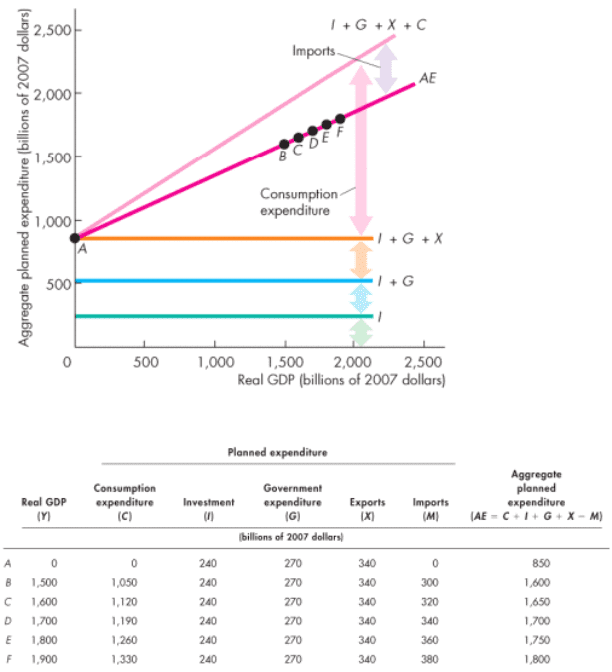Class Notes (1,100,000)
CA (620,000)
Western (60,000)
ECON (1,000)
Lecture 8

# Economics 1022A/B Lecture Notes - Lecture 8: Consumption Function, Chapter 27, Aggregate Demand

Department
Economics
Course Code
ECON 1022A/B
Professor
Jeannie Gillmore
Lecture
8

This preview shows page 1. to view the full 5 pages of the document.
Several factors influence consumption expenditure and saving.
The most direct influence is disposable income, which is real GDP or aggregate income minus net
taxes (taxes minus transfer payments).
Planned consumption expenditure plus planned saving equals disposable income.
The greater the disposable income, the greater is consumption expenditure and the greater is saving.
The relationship between consumption expenditure and disposable income, other things remaining
the same, is called the consumption function.
The relationship between saving and disposable income, other things remaining the same, is called
the saving function. 2 3
The extent to which a change in disposable income changes consumption expenditure depends on
the marginal propensity to consume.
The marginal propensity to consume (MPC) is the fraction of a change in disposable income that is
consumed.
The marginal propensity to consume is calculated as the change in consumption expenditure ΔC,
divided by the change in disposable income, ΔYD.
MPC = ΔC ÷ ΔYD
That is:
The extent to which a change in disposable income changes saving depends on the marginal
propensity to save.
The marginal propensity to save is calculated as the change in saving ΔS, divided by the change in
disposable income, ΔYD.
MPS = ΔS ÷ ΔYD
That is:
The marginal propensity to consume plus the marginal propensity to save sum to 1.
C + S = YD
ΔC + ΔS = ΔYD (ΔC ÷ ΔYD) + (ΔS ÷ ΔYD) = (ΔYD ÷ ΔYD)
MPC + MPS = 1
You can see this from the following:
The figure shows the MPC as the slope of the consumption function and the MPS as the slope of the
saving function.
MPC is \$150 billion ÷ \$200 billion = 0.75.
MPS is \$50 billion ÷ \$200 billion = 0.25.
The marginal propensity to import is the fraction of an increase in real GDP that is spent on
imports.
It is calculated as the change in imports divided by the change in real GDP that brought it
about, other things remaining the same.
The relationship between imports and real GDP is determined by the marginal propensity to import.
Fixed Prices and Expenditure Plans:
Aggregate planned expenditure equals planned consumption expenditure plus planned investment
plus planned government expenditures plus planned exports minus planned imports.
The table on the next page sets out an aggregate expenditure schedule, together with the
components of aggregate planned expenditure.
The figure shows the AE curve.
It is made up from the consumption function minus the import function plus I, G, and X.
Autonomous Expenditure
Induced Expenditure
The AE curve can be thought of as two parts:
Autonomous Expenditure is the sum of investment, government expenditures, and exports, which
does not vary with real GDP
Induced Expenditure is consumption expenditure minus imports, which varies with real GDP
Real GDP with a Fixed Price Level
Lecture 8 - Chapter 27: Expenditure Multipliers
March 12, 2017
10:33 AM
Lecture Notes Page 1
###### You're Reading a Preview

Unlock to view full version

Only page 1 are available for preview. Some parts have been intentionally blurred.The figure shows equilibrium expenditure
The 45-degree line shows actual aggregate expenditure at each level of real GDP.
Only at point D is actual aggregate expenditure equal to aggregate planned expenditure.
So, \$1,700 billion is equilibrium real GDP.
Below \$1,700 billion, aggregate planned expenditure exceeds real GDP.
Above \$1,700 billion, aggregate planned expenditure is less than real GDP.
The bottom figure shows the unplanned inventory changes that bring a convergence to equilibrium
expenditure.
Below \$1,700 billion, aggregate planned expenditure exceeds real GDP, so inventories fall below their
target levels.
Firms increase production.
Real GDP increases.
Above \$1,700 billion, aggregate planned expenditure is less than real GDP, so inventories rise above
their target levels. 7
Firms decrease production.
Real GDP decreases.
At \$1,700 billion, aggregate planned expenditure equals real GDP, so inventories remain at their
target levels.
Firms do not change production.
Real GDP remains constant.
A change in expenditure generates a change in income.
The change in income induces a change in consumption expenditure.
The change in consumption expenditure increases income further.
Where does the process end?
The multiplier is the amount by which a change in autonomous expenditure is magnified or
multiplied to determine the change in equilibrium expenditure and real GDP.
The figure shows equilibrium expenditure.
The AE curve shows aggregate planned expenditure at each level of real GDP.
By studying the schedule, you can work out the equilibrium of real GDP (Y) and aggregate
expenditure (AE)
The Multiplier
Lecture Notes Page 2
###### You're Reading a Preview

Unlock to view full version